# Underwater Target Detection with an Active Sonar System

This example shows how to simulate an active monostatic sonar scenario with two targets. The sonar system consists of an isotropic projector array and a single hydrophone element. The projector array is spherical in shape. The backscattered signals are received by the hydrophone. The received signals includes both direct and multipath contributions.

### Underwater Environment

Multiple propagation paths are present between the sound source and target in a shallow water environment. In this example, five paths are assumed in a channel with a depth of 100 meters and a constant sound speed of 1520 m/s. Use a bottom loss of 0.5 dB in order to highlight the effects of the multiple paths.

Define the properties of the underwater environment, including the channel depth, the number of propagation paths, the propagation speed, and the bottom loss.

```numPaths = 5; propSpeed = 1520; channelDepth = 100; isopath{1} = phased.IsoSpeedUnderwaterPaths(... 'ChannelDepth',channelDepth,... 'NumPathsSource','Property',... 'NumPaths',numPaths,... 'PropagationSpeed',propSpeed,... 'BottomLoss',0.5,... 'TwoWayPropagation',true); isopath{2} = phased.IsoSpeedUnderwaterPaths(... 'ChannelDepth',channelDepth,... 'NumPathsSource','Property',... 'NumPaths',numPaths,... 'PropagationSpeed',propSpeed,... 'BottomLoss',0.5,... 'TwoWayPropagation',true); ```

Next, create a multipath channel for each target. The multipath channel propagates the waveform along the multiple paths. This two-step process is analogous to designing a filter and using the resulting coefficients to filter a signal.

```fc = 20e3; % Operating frequency (Hz) channel{1} = phased.MultipathChannel(... 'OperatingFrequency',fc); channel{2} = phased.MultipathChannel(... 'OperatingFrequency',fc); ```

### Sonar Targets

The scenario has two targets. The first target is more distant but has a larger target strength, and the second is closer but has a smaller target strength. Both targets are isotropic and stationary with respect to the sonar system.

```tgt{1} = phased.BackscatterSonarTarget(... 'TSPattern',-5*ones(181,361)); tgt{2} = phased.BackscatterSonarTarget(... 'TSPattern',-15*ones(181,361)); tgtplat{1} = phased.Platform(... 'InitialPosition',[500; 1000; -70],'Velocity',[0; 0; 0]); tgtplat{2} = phased.Platform(... 'InitialPosition',[500; 0; -40],'Velocity',[0; 0; 0]); ```

The target positions, along with the channel properties, determine the underwater paths along which the signals propagate. Plot the paths between the sonar system and each target. Note that the z-coordinate determines depth, with zero corresponding to the top surface of the channel, and the distance in the x-y plane is plotted as the range between the source and target.

```helperPlotPaths([0;0;-60],[500 500; 1000 0; -70 -40], ... channelDepth,numPaths) ```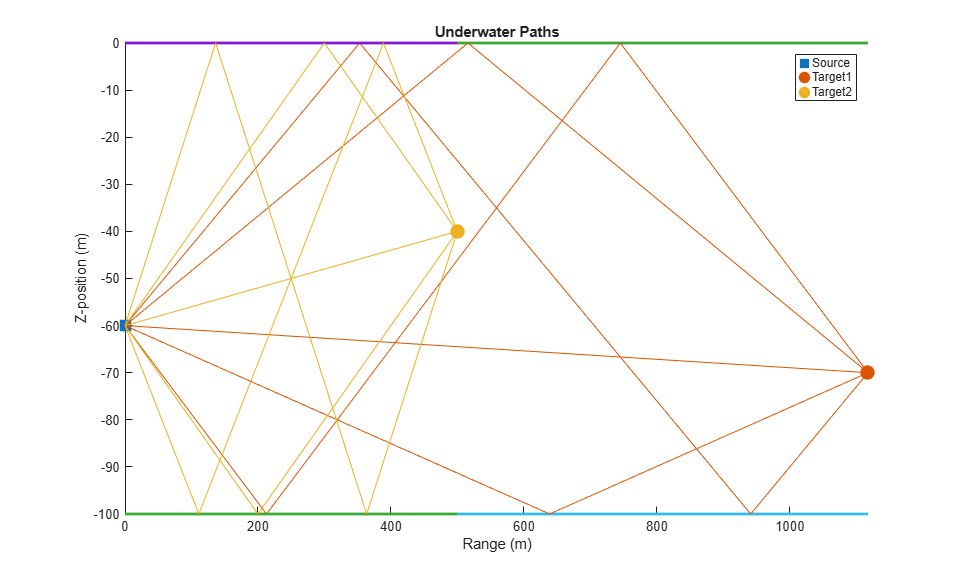Transmitted Waveform

Next, specify a rectangular waveform to transmit to the targets. The maximum target range and desired range resolution define the properties of the waveform.

```maxRange = 5000; % Maximum unambiguous range rangeRes = 10; % Required range resolution prf = propSpeed/(2*maxRange); % Pulse repetition frequency pulse_width = 2*rangeRes/propSpeed; % Pulse width pulse_bw = 1/pulse_width; % Pulse bandwidth fs = 2*pulse_bw; % Sampling rate wav = phased.RectangularWaveform(... 'PulseWidth',pulse_width,... 'PRF',prf,... 'SampleRate',fs); ```

Update the sample rate of the multipath channel with the transmitted waveform sample rate.

```channel{1}.SampleRate = fs; channel{2}.SampleRate = fs; ```

Transmitter

The transmitter consists of a hemispherical array of back-baffled isotropic projector elements. The transmitter is located 60 meters below the surface. Create the array and view the array geometry.

```plat = phased.Platform(... 'InitialPosition',[0; 0; -60],... 'Velocity',[0; 0; 0]); proj = phased.IsotropicProjector(... 'FrequencyRange',[0 30e3],'VoltageResponse',80,'BackBaffled',true); [ElementPosition,ElementNormal] = helperSphericalProjector(8,fc,propSpeed); projArray = phased.ConformalArray(... 'ElementPosition',ElementPosition,... 'ElementNormal',ElementNormal,'Element',proj); viewArray(projArray,'ShowNormals',true); ```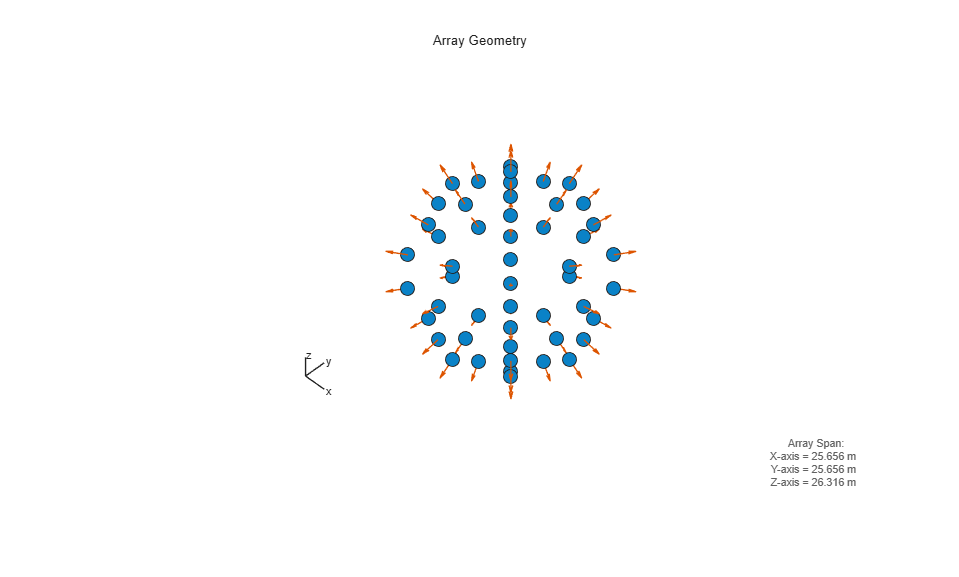View the pattern of the array at zero degrees in elevation. The directivity shows peaks in azimuth corresponding to the azimuth position of the array elements.

```pattern(projArray,fc,-180:180,0,'CoordinateSystem','polar',... 'PropagationSpeed',propSpeed); ```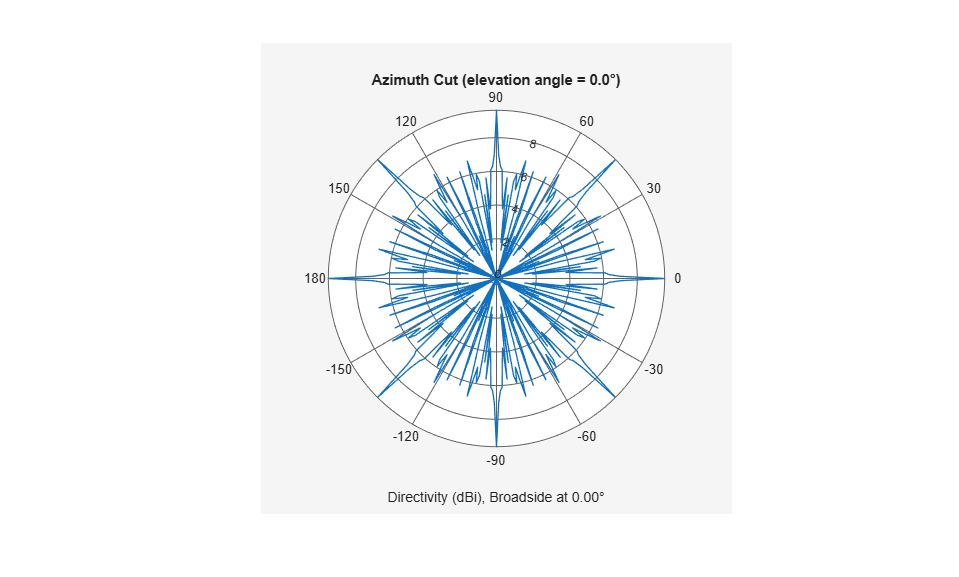The receiver consists of a hydrophone and an amplifier. The hydrophone is a single isotropic element and has a frequency range from 0 to 30 kHz, which contains the operating frequency of the multipath channel. Specify the hydrophone voltage sensitivity as -140 dB.

```hydro = phased.IsotropicHydrophone(... 'FrequencyRange',[0 30e3],'VoltageSensitivity',-140); ```

Thermal noise is present in the received signal. Assume that the receiver has 20 dB of gain and a noise figure of 10 dB.

```rx = phased.ReceiverPreamp(... 'Gain',20,... 'NoiseFigure',10,... 'SampleRate',fs,... 'SeedSource','Property',... 'Seed',2007); ```

In an active sonar system, an acoustic wave is propagated to the target, scattered by the target, and received by a hydrophone. The radiator generates the spatial dependence of the propagated wave due to the array geometry. Likewise, the collector combines the backscattered signals received by the hydrophone element from the far-field target.

```radiator = phased.Radiator('Sensor',projArray,'OperatingFrequency',... fc,'PropagationSpeed',propSpeed); collector = phased.Collector('Sensor',hydro,'OperatingFrequency',fc,... 'PropagationSpeed',propSpeed); ```

### Sonar System Simulation

Next, transmit the rectangular waveform over ten repetition intervals and simulate the signal received at the hydrophone for each transmission.

```x = wav(); % Generate pulse xmits = 10; rx_pulses = zeros(size(x,1),xmits); t = (0:size(x,1)-1)/fs; for j = 1:xmits % Update target and sonar position [sonar_pos,sonar_vel] = plat(1/prf); for i = 1:2 %Loop over targets [tgt_pos,tgt_vel] = tgtplat{i}(1/prf); % Compute transmission paths using the method of images. Paths are % updated according to the CoherenceTime property. [paths,dop,aloss,tgtAng,srcAng] = isopath{i}(... sonar_pos,tgt_pos,... sonar_vel,tgt_vel,1/prf); % Compute the radiated signals. Steer the array towards the target. tsig = radiator(x,srcAng); % Propagate radiated signals through the channel. tsig = channel{i}(tsig,paths,dop,aloss); % Target tsig = tgt{i}(tsig,tgtAng); % Collector rsig = collector(tsig,srcAng); rx_pulses(:,j) = rx_pulses(:,j) + ... rx(rsig); end end ```

Plot the magnitude of non-coherent integration of the received signals to locate the returns of the two targets.

```figure rx_pulses = pulsint(rx_pulses,'noncoherent'); plot(t,abs(rx_pulses)) grid on xlabel('Time (s)') ylabel('Amplitude (V)') title('Integrated Received Pulses') ```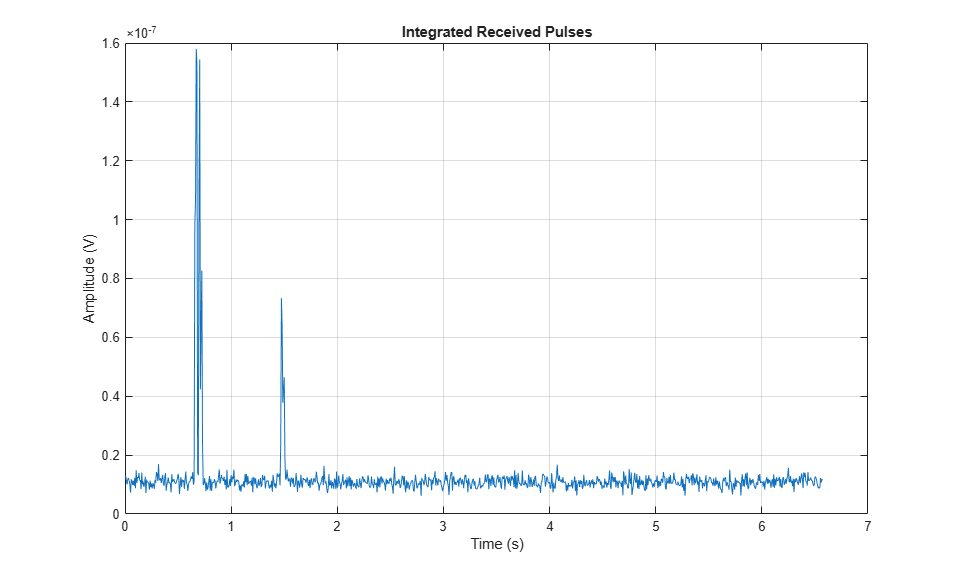The targets, which are separated a relatively large distance, appear as distinct returns. Zoom in on the first return.

```xlim([0.55 0.85]) ```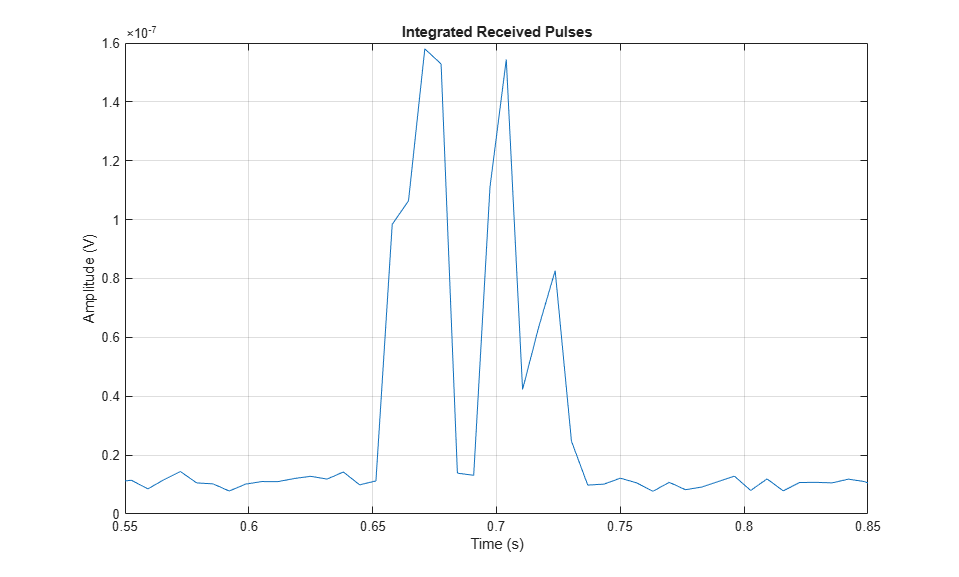The target return is the superposition of pulses from multiple propagation paths, resulting in multiple peaks for each target. The resulting peaks could be misinterpreted as additional targets.

### Active Sonar with Bellhop

In the previous section, the sound speed was constant as a function of channel depth. In contrast, a ray tracing program like Bellhop can generate acoustic paths for spatially-varying sound speed profiles. You can use the path information generated by Bellhop to propagate signals via the multipath channel. Simulate transmission between an isotropic projector and isotropic hydrophone in a target-free environment with the 'Munk' sound speed profile. The path information is contained in a Bellhop arrival file (MunkB_eigenray_Arr.arr).

Bellhop Configuration

In this example, the channel is 5000 meters in depth. The source is located at a depth of 1000 meters and the receiver is located at a depth of 800 meters. They are separated by 100 kilometers in range. Import and plot the paths computed by Bellhop.

```[paths,dop,aloss,rcvAng,srcAng] = helperBellhopArrivals(fc,6,false); helperPlotPaths('MunkB_eigenray') ```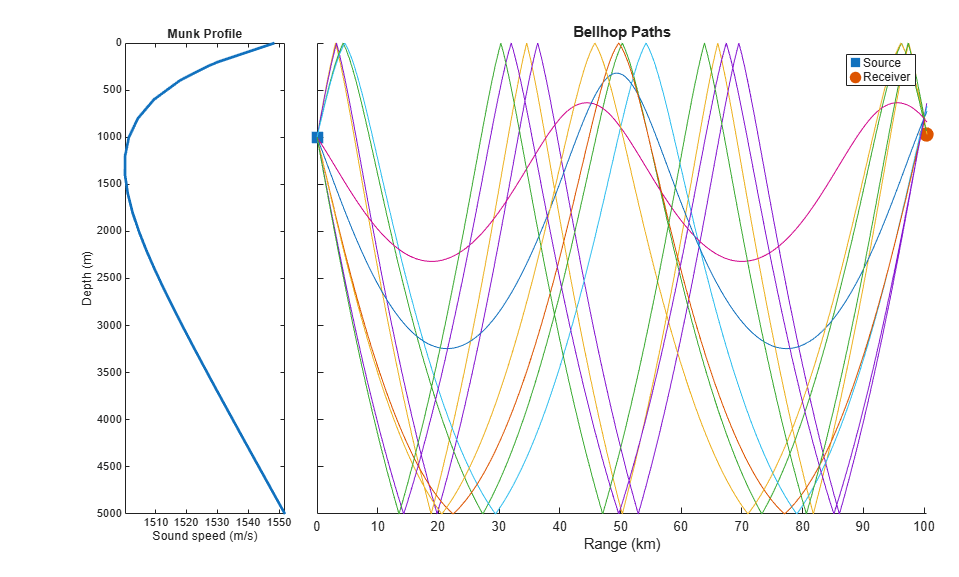For this scenario, there are two direct paths with no interface reflections, and eight paths with reflections at both the top and bottom surfaces. The sound speed in the channel is lowest at approximately 1250 meters in depth, and increases towards the top and bottom of the channel, to a maximum of 1550 meters/second.

Create a new channel and receiver to use with data from Bellhop.

```release(collector) channelBellhop = phased.MultipathChannel(... 'SampleRate',fs,... 'OperatingFrequency',fc); rx = phased.ReceiverPreamp(... 'Gain',10,... 'NoiseFigure',10,... 'SampleRate',fs,... 'SeedSource','Property',... 'Seed',2007); ```

Specify a pulse for the new problem configuration.

```maxRange = 150000; % Maximum unambiguous range prf = propSpeed/(maxRange); % Pulse repetition frequency pulse_width = 0.02; wav = phased.RectangularWaveform(... 'PulseWidth',pulse_width,... 'PRF',prf,... 'SampleRate',fs); ```

Bellhop Simulation

Next, simulate the transmission of ten pulses from transmitter to receiver.

```x = repmat(wav(),1,size(paths,2)); xmits = 10; rx_pulses = zeros(size(x,1),xmits); t = (0:size(x,1)-1)/fs; for j = 1:xmits % Projector tsig = x.*proj(fc,srcAng)'; % Propagate radiated signals through the channel. tsig = channelBellhop(tsig,paths,dop,aloss); % Collector rsig = collector(tsig,rcvAng); rx_pulses(:,j) = rx_pulses(:,j) + ... rx(rsig); end ```

Plot the non-coherent integration of the transmitted pulses.

```figure rx_pulses = pulsint(rx_pulses,'noncoherent'); plot(t,abs(rx_pulses)) grid on xlim([66 70]) xlabel('Time (s)') ylabel('Amplitude (V)') title('Integrated Received Pulses') ```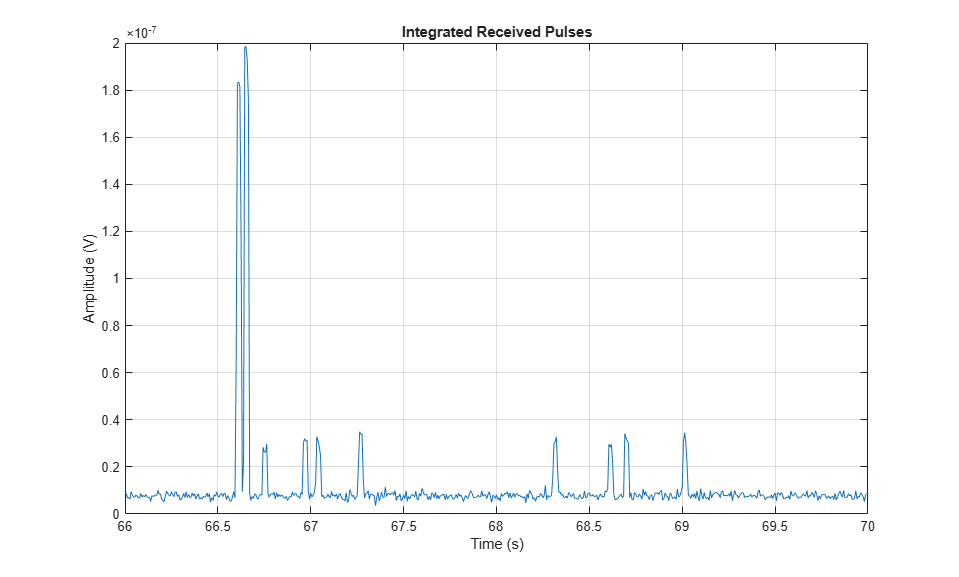The transmitted pulses appear as peaks in the response. Note that the two direct paths, which have no interface reflections, arrive first and have the highest amplitude. In comparing the direct path received pulses, the second pulse to arrive has the higher amplitude of the two, indicating a shorter propagation distance. The longer delay time for the shorter path can be explained by the fact that it propagates through the slowest part of the channel. The remaining pulses have reduced amplitude compared to the direct paths due to multiple reflections at the channel bottom, each contributing to the loss.

### Summary

In this example, acoustic pulses were transmitted and received in shallow-water and deep-water environments. Using a rectangular waveform, an active sonar system detected two well-separated targets in shallow water. The presence of multiple paths was apparent in the received signal. Next, pulses were transmitted between a projector and hydrophone in deep water with the 'Munk' sound speed profile using paths generated by Bellhop. The impact of spatially-varying sound speed was noted.

### Reference

Urick, Robert. Principles of Underwater Sound. Los Altos, California: Peninsula Publishing, 1983.